##### Multicollinearity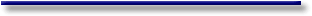# Online Econometrics Textbook - Regression Extensions - Multicollinearity - Detection of Multicollinearity

#### III.IV.1 Detection of multicollinearity

Since we have seen that strong linear associations between exogenous variables are not always catastrophic, it seems that good detection measures for "bad" multicollinearity are no spurious luxury.

Let us have a look at some possible diagnostics (or symptoms).

##### 1. correlation matrix between exogenous variables

It is obvious that the linear correlations are the easiest detection diagnostic available to the researcher. Though it should be noted that this is no good measure for "bad" multicollinearity, as stated above.##### 2. high R-square and low T-Stats

This criterion, like the previous, doesn't give us clear-cut answers with respect to the harmfulness of multicollinearity.

##### 3. high R-square and low quadratic partial correlations between an exogenous variable and the dependent variable

By definition, a partial correlation coefficient between a variable X (exogenous) and another variable Y (endogenous), given that a third variable Z (exogenous) remains constant, is(III.IV.1-1)

where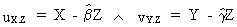(III.IV.1-2)Clearly this measure is much better than the previous ones. Above that, one may introduce more than just one exogenous variable Z, which is held constant.

```Correlation matrix:

rho(  employ.dba(-0),    ship.dba(-0)) = +0.9344

rho(  expend.dba(-0),    ship.dba(-0)) = +0.9055

rho(  expend.dba(-0),  employ.dba(-0)) = +0.8467

Partial Correlation matrix:

phi(  employ.dba(-0),    ship.dba(-0)) = +0.7429

phi(  expend.dba(-0),    ship.dba(-0)) = +0.6034

phi(  expend.dba(-0),  employ.dba(-0)) = +0.0037

```

Observe how in the above example the partial correlation between expend and ship is much lower than the correlation coefficient.

##### 4. eigenvalues

First compute the eigenvalues of the matrix X'X. In case of linear dependence between the variables the eigenvalues of all different eigenvectors will differ much from each other, such that the ratio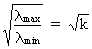(III.IV.1-3)

becomes quite large. If the square root of k (c.q. the condition number) is much larger than (approx.) 30 this could be, according to many authors, a sign of harmful multicollinearity.

The variance of the OLS regression parameters can be shown to be equal to the residual variance multiplied by the sum of the variance proportions of all eigenvalues. This so-called Variance Decomposition Analysis (Belsley, Kuh, and Welsch) gives us much more detailed information.

Below you ll find an example of how the condition index and the Variance Decomposition Analysis can be used to test for possible multicollinearity (this test is applied to our example-equation):

```Variance Decomposition Analysis:

computing X'X and trace

trace = 1877722.

compare convergence 853155.2309 versus 0.8531552309

compare convergence 284385.077 versus 0.8531552309

compare convergence 94795.02565 versus 0.8531552309

compare convergence 31598.34188 versus 0.8531552309

compare convergence 10532.78063 versus 0.8531552309

compare convergence 3510.926876 versus 0.8531552309

compare convergence 1170.308959 versus 0.8531552309

compare convergence 390.1029862 versus 0.8531552309

compare convergence 130.0343287 versus 0.8531552309

compare convergence 43.34477625 versus 0.8531552309

compare convergence 14.44825875 versus 0.8531552309

compare convergence 4.81608625 versus 0.8531552309

compare convergence 1.605362083 versus 0.8531552309

compare convergence 0.5351206944 versus 0.8531552309

```
```Variance decomp. proportions

```
```eigen value     V(b1)           V(b2)           V(b3)           condition index

40686.6875      0.8411622123    0.7794250194    3.233608407e-00 6.719400896 OK

1837018.116     3.372416044e-00 9.537926949e-00 4.356289766e-01 1. OK

17.19699145     0.1554653717    0.1251957111    0.9999999677    326.8364479 > 30

```
##### 5. determinants

When the matrix X'X contains columns or rows which are linearly dependent from each other, we call this matrix singular (and can therefore not invert it). If the matrix is only almost singular, the process of inverting yields a lot of (rounding-off-)errors.

Therefore it is good to compute the determinant of X'X (without constant term): if the determinant is equal to zero, this indicates perfect multicollinearity; if the determinant is small then this is an in­dication of an almost singular matrix.

Also note that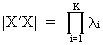(III.IV.1-4)

Obviously this diagnostic is a very weak measure for "bad" (harmful) multicollinearity. It would be better to use a measure which reflects the sensitivity of the parameters with respect to small changes in X'X.

For this reason the author prefers a self-developed measure defined by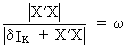(III.IV.1-5)

where d is a small number and w is the measure.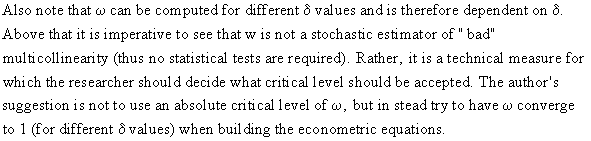##### 6. help regressions

It is evident to see that multicollinearity always exists in case the regressors influence each other. This can be measured by the R-square of a regression of an arbitrary exogenous variable with respect to all other K - 1 exogenous variables (constant included).

The higher the R-square, the higher the degree of multicollinearity.

The respective F-statistic may be calculated by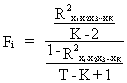(III.IV.1-6)

The same information might be expressed in terms of the so-called Variance Inflation Factors:As the name indicates, the VIF measures the factor by which the parameters variance (in an orthogonal regression; hence without multicollinearity) is multiplied (c.q. inflated).

Below you ll find an example of how the condition index and the Variance Inflation Factors can be used to test for possible multicollinearity (this test is applied to our example-equation):

VIF(variable 1) = 13.48449151
VIF(variable 2) = 3.536336574

(Note: the VIF of the constant term is never computed, since it is meaningless.)

##### 7. sensitivity of parameters

Under the assumptions of the general linear statistical model the author also suggests comparing parameters of simple and multiple regressions. It has been shown in eq. (II.II.1-27) that in case of zero multicollinearity simple regression yields the same parameter estimation than multiple regression. The multicollinearity problem is proportional to the sensitivity of the parameters with respect to the introduction of new exogenous variables. This can thus be used for detection of possible multicollinearity, though it must be kept in mind that simple regression (probably) suffers more from the UVB than multiple regression.

Based on this concept, the author has developed an (unpublished) algorithm to compute a measure of uncertainty induced by the presence of more than just one exogenous variables. The output of this so-called Bias Decomposition Analysis consists of a variance measure which can be shown to be caused ONLY by multiple exogenous variables, and NOT by the number of observations.No news at the moment...© 2000-2018 All rights reserved. All Photographs (jpg files) are the property of Corel Corporation, Microsoft and their licensors. We acquired a non-transferable license to use these pictures in this website.
The free use of the scientific content in this website is granted for non commercial use only. In any case, the source (url) should always be clearly displayed. Under no circumstances are you allowed to reproduce, copy or redistribute the design, layout, or any content of this website (for commercial use) including any materials contained herein without the express written permission.

Information provided on this web site is provided "AS IS" without warranty of any kind, either express or implied, including, without limitation, warranties of merchantability, fitness for a particular purpose, and noninfringement. We use reasonable efforts to include accurate and timely information and periodically updates the information without notice. However, we make no warranties or representations as to the accuracy or completeness of such information, and it assumes no liability or responsibility for errors or omissions in the content of this web site. Your use of this web site is AT YOUR OWN RISK. Under no circumstances and under no legal theory shall we be liable to you or any other person for any direct, indirect, special, incidental, exemplary, or consequential damages arising from your access to, or use of, this web site.

Contributions and Scientific Research: Prof. Dr. E. Borghers, Prof. Dr. P. Wessa
Please, cite this website when used in publications: Xycoon (or Authors), Statistics - Econometrics - Forecasting (Title), Office for Research Development and Education (Publisher), http://www.xycoon.com/ (URL), (access or printout date).